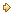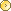Visitors Online: 77 | Monday 14th October 2019

CBSE Guess > Papers > Question Papers > Class XII > 2006 > Economics >Compartment Delhi Set - I

Economics — 2006 (Set I — Compartment Delhi)

SECTION - A

Q. 1. Answer the following questions: (1 x 4 = 4)

1. Give meaning of opportunity cost.
2. Define marginal physical product.
3. Give meaning of supply.
4. Define fixed costs.

Q. 2. Draw and define production possibility curve. Why is it downward sloping from left to right? (3)

For Blind Candidates only in lieu of Q. No. 2
Explain the problem of ‘what to produce’.

Q. 3. Define utility. Explain briefly the law of diminishing marginal utility. (3)

Q. 4. State clearly any three features of a perfectly competitive market. (3)

Q. 5. Explain ‘differentiated products’ characteristic of monopolistic competition. (3)

Q. 6. Demand of a product is ‘elastic’. Its price falls. What will be its effect on total expenditure on the product? Give a numerical example. (4)

Q. 7. A firm sells 1000 units of a product at a price of Rs. 10 per unit. Its price elasticity of supply is 3. How many units will the firm be able to sell if the price falls to Rs. 7.50 per unit? (4)

Q. 8. Identify different phases of the law of variable proportions from the following schedule. Give reasons for your answer. (4)

 Variable Input (Units) Total Physical Product (Units) 1 2 3 4 5 4 9 13 15 12

Q. 9. Explain the changes that take place when at a given price of a commodity there is excess supply of it. Use diagram.

Or

A product market is in equilibrium. Suppose the demand for the product decreases. What changes will take place in the market? Use diagram. (4)

For Blind Candidates only in lieu of Q. No. 9

At a given price of a commodity there is excess supply of it. Is this price an equilibrium price ? If not, how is the equilibrium price reached, explain. (4)

Or

Explain the effects of an increase in demand of a commodity on its equilibrium price and equilibrium quantity.

Q. 10. Explain the effects of increase in income of the buyers of good ‘X’ cm the demand for ‘X’ use diagram showing demand for good on the x-axis and its price on the y-axis. (6)

Or

A consumer consumes good ‘X’. Explain the effects of fall in prices of related goods on the demand of ‘X’. Use diagram showing demand for good ‘X’ on the x-axis and its price on the y - axis.

For Blind Candidates only in lieu of Q. No. 10

Explain the effects of change in the income of the buyers of a good on its demand. (6)

Or

Explain the effects of change in the prices of related goods on the demand of a given good.

Q. 11. Explain briefly the following determinants of supply: (6)

1. Increase in the prices of inputs
2. Decrease in tax on the product
3. Technological change

Q. 12. Draw Average Total Cost, Average Variable Cost and Mar ginal Cost curves in a single graph. Also explain the relation between Marginal Cost and Average Total Cost. (6)

SECTION - B

Q. 13. Answer the following questions : (1 x 4 = 4)

1. Define macroeconomics.
2. Give one example each of mieroeconomics and macroeconomics.
3. What does balance of payments account record?
4. What is the balance of visible items in the balance of payments accounts called?

Q. 14. Calculate Net Value Added at Market Price from the following data: (3)

 (Rs. crores) Depreciation Sales Opening stock Intermediate consumption Excise duty Change in stocks 5 100 20 70 10 (—) 10

Q. 15. In an economy investment increases by Rs.120 crores. The value of investment multiplier is 4. Calculate the marginal propensity to consume. (3)

Q. 16. What is deflationary gap ? State two measures to remove it. (3)

Q. 17. Explain how foreign exchange rate is determined in the market. (3)

Q. 18. Explain the ‘medium of exchange’ function of money. (4)

Or

Explain the ‘store of value’ function of money.

Q. 19. Describe the ‘banker to the government’ function of the central bank. (4)

Q. 20. Define a government budget. State its any three objectives. (4)

Q. 21. What does fiscal deficit in a government budget mean? What are its implications?(4)

Q. 22. Explain the following terms giving suitable examples: (6)

1. Final products
2. Intermediate expenditure
3. Transfer payment

Q. 23. Explain the roles of

1. bank rate policy and
2. changes in government expenditure in reducing ‘excess demand’ in an economy. (6)

Or

Explain the roles of

1. open market operations and
2. changes in government expenditure in solving the problem of ‘deficient demand’ in an economy.

Q. 24. Calculate

1. Net National Disposable Income and
2. Private Income from the following data: (2, 4)
 ( RS. Crores) Net indirect tax Compensfttion of employees Personal taxes Operating surplus Corporate profit tax Mixed income of the self-employed National debt interest Savings of non-departmental enterprises Current transfers from government Income from property and entrepreneurship accruing to the government administrative departments Net current transfers to the rest of the world  Net factor income from abroad 90 400 100 200 80 500 70 40 60 30 20 (- 50)

 Economics 2006 Question Papers Class XII Delhi Outside Delhi Compartment Delhi Compartment Outside DelhiSet ISet ISet ISet I

 CBSE 2006 Question Papers Class XIIEnglishSociologyFunctional EnglishPsychologyMathematicsPhilosopyPhysicsComputer ScienceChemistryEntrepreneurshipBiologyInformatics PracticesGeographyMultimedia & Web TechnologyEconomicsBiotechnologyBusiness StudiesPhysical EducationAccountancyFine ArtsPolitical ScienceHistoryAgriculture